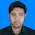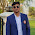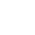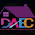### Constructor, Inheritance and Polymorphism programming practice in C++

.
Write a program to check a number is Even or Not using Constructor.

Find the Area of a Rectangle, circle and Triangle using Inheritance.

Write any program to call a member function which will be cause of a different function to be executed depending on the types of object that invokes the function.

1.Name: Md. Johorul Islam
ID: 201720237
CSE-DIP-55 Batch
---------------------
problem 1 and 2 solved. can't understand problem 3.

2.Name:Asib Bin Rhaman.
ID:201730160
Dept:CSE(Diploma)
Batch:56th
Campus:Uttara.
----------------------
# Write a program to check a number is Even
or Not using Constructor.

#include
using namespace std;
class myClass
{
public:
myClass(int num)
{
if(num%2 == 0){
cout<
using namespace std;
class circle
{
public:
circle(int x)
{
}
double area()
{
}
};
int main()
{
cout<<"Area of this circle = "<
using namespace std;
class triangle
{
public:
int base, height;
triangle(int x, int y)
{
base = x;
height = y;
}
double area()
{
return 0.5*base*height;;
}
};
int main()
{
int base, height;
cout<<"Input triangle base = ";
cin>>base;
cout<<"Input triangle height = ";
cin>>height;
triangle obj(base, height);
cout<<"Area of this triangle = "<
using
namespace std;
class ageCalc
{
public:
int birth, year = 2018;
ageCalc(int x)
{
birth = x;
age();
}
void age()
{
ageCalc obj(birth);
}

output:
Which year do you born 1993
You are about 25 years old

3.CSE-55th Batch
Roll: 201720297

https://pastebin.com/v5yuWpqi

4.Name: Md. Johorul Islam
ID: 201720237
CSE-DIP-55 Batch
---------------------
problem 3 solution.

5.Fazle rabbi khan taron
201720263
55th

#include
using namespace std;

class Shape {
protected:
int width, height;

public:
Shape( int a = 0, int b = 0){
width = a;
height = b;
}
int area() {
cout << "Parent class area :" <<endl;
return 0;
}
};
class Rectangle: public Shape {
public:
Rectangle( int a = 0, int b = 0):Shape(a, b) { }

int area () {
cout << "Rectangle class area :" <<endl;
return (width * height);
}
};

class Triangle: public Shape {
public:
Triangle( int a = 0, int b = 0):Shape(a, b) { }

int area () {
cout << "Triangle class area :" <<endl;
return (width * height / 2);
}
};

class Rectangle
{
int l,b;
Rectangle(int x,int y)
{
l=x;
b=y;
}
int getRectangle()
{
return l*b;
}
}
class Triangle extends Rectangle
{
float a;
Triangle(int v,int u)
{
super(u,v);
}
float getTriangle()
{
a=(float)l/2*l*b;
return (a);
}
}
class RectangleTriangle
{
public static void main(String args[])
{
Triangle m=new Triangle(500,80);
System.out.println("Area of Rectangle is : " +m.getRectangle());
System.out.println("Area of Triangle is : "+m.getTriangle());
}
}

import java.util.Scanner;

class OddOrEven
{
public static void main(String args[])
{
int x;
System.out.println("Enter an integer to check if it is odd or even ");
Scanner in = new Scanner(System.in);
x = in.nextInt();

if ( x % 2 == 0 )
System.out.println("You entered an even number.");
else
System.out.println("You entered an odd number.");
}
}

6.Name: Jibon Chandra Roy
ID: 201730589
CSE-DIP-56 Batch

https://www.dropbox.com/s/1ok1rgn0kr6mnll/Assignment.pdf?dl=0

7.Name: Md.Toufiqur Rahman
ID: 201730027
CSE-DIP-56 Batch

--------------------

8.Minhazul Abid Sakin
roll: 201720265
batch: 55th

9.Name: Sayeka Jannat
ID: 201720097
CSE-DIP-55 Batch

1. Write a program to check a number is Even or Not using Constructor.

#include
using namespace std;

class exam
{
int n;
public:
exam()
{
cout<<"Enter a number: \n";
cin>>n;
if(n%2==0)
cout<<" This is a EVEN number ";
else
cout<<"This is a Not a even number";
}
};

int main()
{
exam();
return 0;
}

2. Find the Area of a Rectangle, circle and Triangle using Inheritance.

#include
using namespace std;

class exam{
public:
int a=4,b=5,c=7;

int ractangle(){
return a*b;
}
};
class date: public exam
{
public:
int triangle()
{
return (a*b/2);}

float circle(){
return 3.14*c*c;}

};
int main()
{
date obj;
obj.ractangle();
obj.triangle();
obj.circle();
cout<<obj.ractangle()<<endl;
cout<<obj.triangle()<<endl;
cout<<obj.circle();
return 0;
}

Comment Here